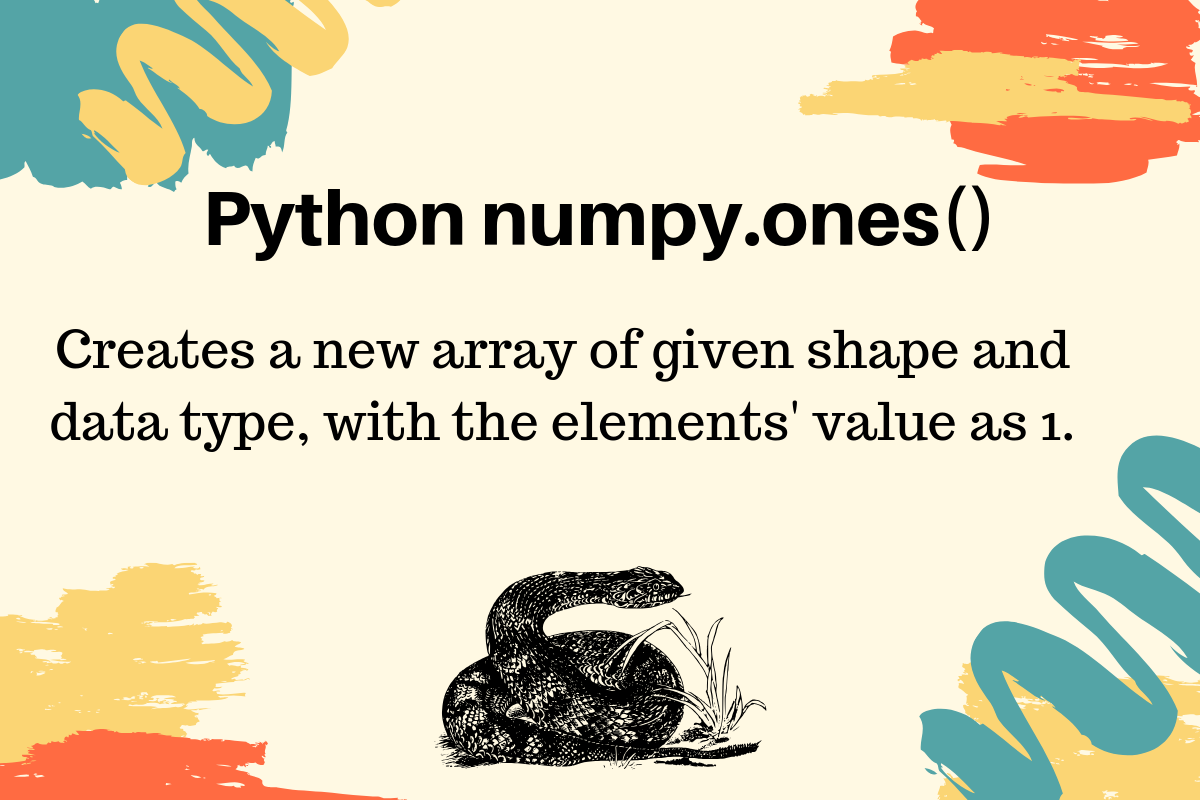# numpy.ones() in Python

Published on August 3, 2022By PankajWhile we believe that this content benefits our community, we have not yet thoroughly reviewed it. If you have any suggestions for improvements, please let us know by clicking the “report an issue“ button at the bottom of the tutorial.

Python numpy.ones() function returns a new array of given shape and data type, where the element’s value is set to 1. This function is very similar to numpy zeros() function.

## numpy.ones() function arguments

The numpy.ones() function syntax is:

``````ones(shape, dtype=None, order='C')
``````
• The shape is an int or tuple of ints to define the size of the array. If we just specify an int variable, a one-dimensional array will be returned. For a tuple of ints, the array of given shape will be returned.
• The dtype is an optional parameter with default value as a float. It’s used to specify the data type of the array, for example, int.
• The order defines the whether to store multi-dimensional array in row-major (C-style) or column-major (Fortran-style) order in memory.

## Python numpy.ones() Examples

Let’s look at some examples of creating arrays using the numpy ones() function.

### 1. Creating one-dimensional array with ones

``````import numpy as np

array_1d = np.ones(3)
print(array_1d)
``````

Output:

``````[1. 1. 1.]
``````

Notice that the elements are having the default data type as the float. That’s why the ones are 1. in the array.

### 2. Creating Multi-dimensional array

``````import numpy as np

array_2d = np.ones((2, 3))
print(array_2d)
``````

Output:

``````[[1. 1. 1.]
[1. 1. 1.]]
``````

### 3. NumPy ones array with int data type

``````import numpy as np

array_2d_int = np.ones((2, 3), dtype=int)
print(array_2d_int)
``````

Output:

``````[[1 1 1]
[1 1 1]]
``````

### 4. NumPy Array with Tuple Data Type and Ones

We can specify the array elements as a tuple and specify their data types too.

``````import numpy as np

array_mix_type = np.ones((2, 2), dtype=[('x', 'int'), ('y', 'float')])
print(array_mix_type)
print(array_mix_type.dtype)
``````

Output:

``````[[(1, 1.) (1, 1.)]
[(1, 1.) (1, 1.)]]
[('x', '<i8'), ('y', '<f8')]
``````

Reference: API Doc

Thanks for learning with the DigitalOcean Community. Check out our offerings for compute, storage, networking, and managed databases.Pankaj

author

#### Still looking for an answer?

Ask a questionSearch for more help

Click below to sign up and get \$200 of credit to try our products over 60 days!

### Popular Topics##### Hollie's Hub for Good

Working on improving health and education, reducing inequality, and spurring economic growth? We’d like to help.##### Become a contributor

You get paid; we donate to tech nonprofits.

##### Welcome to the developer cloud

DigitalOcean makes it simple to launch in the cloud and scale up as you grow – whether you’re running one virtual machine or ten thousand.© 2023 DigitalOcean, LLC.